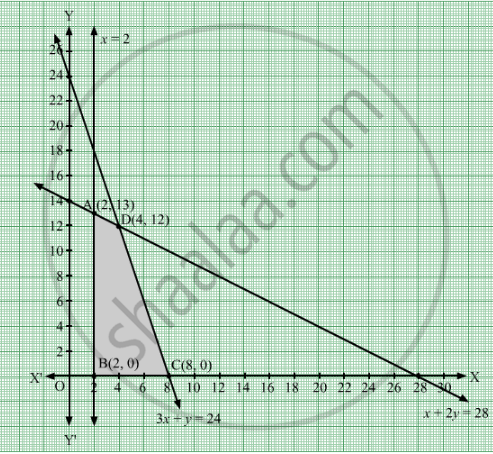# Solve the Following Lpp Graphically: Maximize Z = 20 X + 10 Y Subject to the Following Constraints X + 2 Y ≤ 28 3x+ Y ≤ 24 X ≥ 2x. Y ≥ 0 - Mathematics

Sum

Solve the following LPP graphically:
Maximize Z = 20 x + 10 y
Subject to the following constraints

$x +$2$y \leq$28
3x+ $y \leq$24
$x \geq$ 2x.
$y \geq$  0

#### Solution

The given constraints are
x + 2y ≤ 28

3x + y ≤ 24
x ≥ 2
xy ≥ 0
Converting the given inequations into equations, we get
x + 2y = 28, 3x + y = 24, x = 2, x = 0 and = 0
These lines are drawn on the graph and the shaded region ABCD represents the feasible region of the given LPP.It can be observed that the feasible region is bounded. The coordinates of the corner points of the feasible region are A(2, 13), B(2, 0), C(4, 12) and D(8, 0).

The values of the objective function, Z at these corner points are given in the following table:

 Corner Point Value of the Objective Function Z = 20x + 10y A(2, 13) Z = 20 × 2 + 10 × 13 = 170 B(2, 0) Z = 20 × 2 + 10 × 0 = 40 C(8, 0) Z = 20 × 8 + 10 × 0 = 160 D(4, 12) Z = 20 × 4 + 10 × 12 = 200

From the table, is maximum at = 4 and y = 12 and the maximum value of is 200.

Thus, the maximum value of Z is 200.

Concept: Graphical Method of Solving Linear Programming Problems
Is there an error in this question or solution?

#### APPEARS IN

RD Sharma Class 12 Maths
Chapter 30 Linear programming
Exercise 30.2 | Q 27 | Page 33